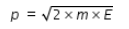# Relationship between kinetic energy and momentum

Relationship between kinetic energy and momentum

Kinetic Energy E = (1/2)×m×v2
where m is mass, v is velocity
2×m×E = (m×v)2
if momentum p = m×v, then